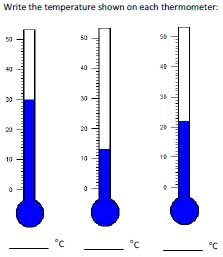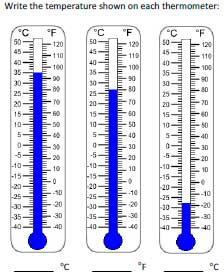# Temperature Worksheets

Temperature worksheets contain reading thermometer, drawing line to show the temperature, comparing between two different temperature units (Celsius and Fahrenheit), converting between Celsius, Fahrenheit and Kelvin, teacher templates and more.Degrees reading from 0 to 50:

Degrees reading from 0 to 100:

Grab 'em All

Degrees reading from -50 to 50:

Simple thermometer worksheets with the scale graduated in Fahrenheit.

Reading from 0 to 50 (increment by 1):

Reading from 0 to 100 (increment by 2):

Grab 'em All

Reading from -50 to 50 (increment by 2):

Compare Celsius and Fahrenheit

The thermometer in these worksheets include both Celsius and Fahrenheit readings along the sides. Read the thermometer and identify the temperature.Comparing Temperatures 2

Comparing Temperatures 3

Shade the thermometers to show the temperatures provided in each worksheet. Color the figure as precise as possible.

Grab 'em All

Grab 'em All

## Convert Between Temperatures

Convert from Celsius to Fahrenheit

To convert Celsius to Fahrenheit, multiply the degree by 1.8 and add 32.

Example: Convert 25°C to °F.
F = 1.8 (25) + 32 = 45 + 32 = 77°F

Convert from Fahrenheit to Celsius

Use the formula below to convert each Fahrenheit degree into Celsius.

C = (F - 32) * (5/9)

Example: Convert 77°F to °C.
C = (77 - 32) * (5/9) = 45 * 5/9 = 225/9 = 25°C

Convert between Temperatures: Mixed

The conversion worksheets include both Celsius and Fahrenheit in each practice sheet.Conversion Worksheet: Easy 2

Convert between Celsius and Kelvin

To convert Celsius to Kelvin: K = C + 273.15

To convert Kelvin to Celsius: C = K - 273.15

Celsius and Kelvin: Easy 2

## Teacher Template

Thermometer Template

Each template includes blank thermometers with readings along the side. Parents and teachers may choose the template they want and set their own questions.

Thermometer Template: 0 to 100

Thermometer Template: -50 to 50

Celsius and Fahrenheit: Combined

The entire collection of worksheets on temperature can be downloaded in a jiffy!

Related Worksheets